# alpenglow.experiments package¶

## alpenglow.experiments.ALSFactorExperiment module¶

class alpenglow.experiments.ALSFactorExperiment.ALSFactorExperiment(dimension=10, begin_min=- 0.01, begin_max=0.01, number_of_iterations=15, regularization_lambda=1e-3, alpha=40, implicit=1, clear_before_fit=1, period_length=86400)[source]

This class implements an online version of the well-known matrix factorization recommendation model [Koren2009] and trains it via Alternating Least Squares in a periodic fashion. The model is able to train on explicit data using traditional ALS, and on implicit data using the iALS algorithm [Hu2008].

Hu2008(1,2,3,4,5)

Hu, Yifan, Yehuda Koren, and Chris Volinsky. “Collaborative filtering for implicit feedback datasets.” Data Mining, 2008. ICDM’08. Eighth IEEE International Conference on. Ieee, 2008.

Parameters
• dimension (int) – The latent factor dimension of the factormodel.

• begin_min (double) – The factors are initialized randomly, sampling each element uniformly from the interval (begin_min, begin_max).

• begin_max (double) – See begin_min.

• number_of_iterations (int) – The number of ALS iterations to perform in each period.

• regularization_lambda (double) – The coefficient for the L2 regularization term. See [Hu2008]. This number is multiplied by the number of non-zero elements of the user-item rating matrix before being used, to achieve similar magnitude to the one used in traditional SGD.

• alpha (int) – The weight coefficient for positive samples in the error formula. See [Hu2008].

• implicit (int) – Valued 1 or 0, indicating whether to run iALS or ALS.

• clear_before_fit (int) – Whether to reset the model after each period.

• period_length (int) – The period length in seconds.

• timeframe_length (int) – The size of historic time interval to iterate over at every batch model retrain. Leave at the default 0 to retrain on everything.

## alpenglow.experiments.ALSOnlineFactorExperiment module¶

class alpenglow.experiments.ALSOnlineFactorExperiment.ALSOnlineFactorExperiment(dimension=10, begin_min=- 0.01, begin_max=0.01, number_of_iterations=15, regularization_lambda=1e-3, alpha=40, implicit=1, clear_before_fit=1, period_length=86400)[source]

Combines ALSFactorExperiment and FactorExperiment by updating the model periodically with ALS and continously with SGD.

Parameters
• dimension (int) – The latent factor dimension of the factormodel.

• begin_min (double) – The factors are initialized randomly, sampling each element uniformly from the interval (begin_min, begin_max).

• begin_max (double) – See begin_min.

• number_of_iterations (double) – Number of times to optimize the user and the item factors for least squares.

• regularization_lambda (double) – The coefficient for the L2 regularization term. See [Hu2008]. This number is multiplied by the number of non-zero elements of the user-item rating matrix before being used, to achieve similar magnitude to the one used in traditional SGD.

• alpha (int) – The weight coefficient for positive samples in the error formula. See [Hu2008].

• implicit (int) – Valued 1 or 0, indicating whether to run iALS or ALS.

• clear_before_fit (int) – Whether to reset the model after each period.

• period_length (int) – The period length in seconds.

• timeframe_length (int) – The size of historic time interval to iterate over at every batch model retrain. Leave at the default 0 to retrain on everything.

• online_learning_rate (double) – The learning rate used in the online stochastic gradient descent updates.

• online_regularization_rate (double) – The coefficient for the L2 regularization term for online update.

• online_negative_rate (int) – The number of negative samples generated after online each update. Useful for implicit recommendation.

## alpenglow.experiments.AsymmetricFactorExperiment module¶

class alpenglow.experiments.AsymmetricFactorExperiment.AsymmetricFactorExperiment(dimension=10, begin_min=- 0.01, begin_max=0.01, learning_rate=0.05, regularization_rate=0.0, negative_rate=20, cumulative_item_updates=True, norm_type='exponential', gamma=0.8)[source]

Implements the recommendation model introduced in [Koren2008].

Paterek2007

Arkadiusz Paterek. „Improving regularized singular value decomposition for collaborative filtering”. In: Proc. KDD Cup Workshop at SIGKDD’07, 13th ACM Int. Conf. on Knowledge Discovery and Data Mining. San Jose, CA, USA, 2007, pp. 39–42.

Koren2008

Koren, Yehuda. “Factorization meets the neighborhood: a multifaceted collaborative filtering model.” Proceedings of the 14th ACM SIGKDD international conference on Knowledge discovery and data mining. ACM, 2008.

Parameters
• dimension (int) – The latent factor dimension of the factormodel.

• begin_min (double) – The factors are initialized randomly, sampling each element uniformly from the interval (begin_min, begin_max).

• begin_max (double) – See begin_min.

• learning_rate (double) – The learning rate used in the stochastic gradient descent updates.

• regularization_rate (double) – The coefficient for the L2 regularization term.

• negative_rate (int) – The number of negative samples generated after each update. Useful for implicit recommendation.

• norm_type (str) – Type of time decay; either “constant”, “exponential” or “disabled”.

• gamma (double) – Coefficient of time decay in the case of norm_type == “exponential”.

## alpenglow.experiments.BatchAndOnlineFactorExperiment module¶

class alpenglow.experiments.BatchAndOnlineFactorExperiment.BatchAndOnlineFactorExperiment(dimension=10, begin_min=- 0.01, begin_max=0.01, batch_learning_rate=0.05, batch_regularization_rate=0.0, batch_negative_rate=70, online_learning_rate=0.05, online_regularization_rate=0.0, online_negative_rate=100, period_length=86400)[source]

Combines BatchFactorExperiment and FactorExperiment by updating the model both in batch and continously.

Parameters
• dimension (int) – The latent factor dimension of the factormodel.

• begin_min (double) – The factors are initialized randomly, sampling each element uniformly from the interval (begin_min, begin_max).

• begin_max (double) – See begin_min.

• batch_learning_rate (double) – The learning rate used in the batch stochastic gradient descent updates.

• batch_regularization_rate (double) – The coefficient for the L2 regularization term for batch updates.

• batch_negative_rate (int) – The number of negative samples generated after each batch update. Useful for implicit recommendation.

• timeframe_length (int) – The size of historic time interval to iterate over at every batch model retrain. Leave at the default 0 to retrain on everything.

• online_learning_rate (double) – The learning rate used in the online stochastic gradient descent updates.

• online_regularization_rate (double) – The coefficient for the L2 regularization term for online update.

• online_negative_rate (int) – The number of negative samples generated after online each update. Useful for implicit recommendation.

## alpenglow.experiments.BatchFactorExperiment module¶

class alpenglow.experiments.BatchFactorExperiment.BatchFactorExperiment(dimension=10, begin_min=- 0.01, begin_max=0.01, learning_rate=0.05, regularization_rate=0.0, negative_rate=0.0, number_of_iterations=3, period_length=86400, timeframe_length=0, clear_model=False)[source]

Batch version of alpenglow.experiments.FactorExperiment.FactorExperiment, meaning it retrains its model periodically nd evaluates the latest model between two training points in an online fashion.

Parameters
• dimension (int) – The latent factor dimension of the factormodel.

• begin_min (double) – The factors are initialized randomly, sampling each element uniformly from the interval (begin_min, begin_max).

• begin_max (double) – See begin_min.

• learning_rate (double) – The learning rate used in the stochastic gradient descent updates.

• regularization_rate (double) – The coefficient for the L2 regularization term.

• negative_rate (int) – The number of negative samples generated after each update. Useful for implicit recommendation.

• number_of_iterations (int) – The number of iterations over the data in model retrain.

• period_length (int) – The amount of time between model retrains (seconds).

• timeframe_length (int) – The size of historic time interval to iterate over at every model retrain. Leave at the default 0 to retrain on everything.

• clear_model (bool) – Whether to clear the model between retrains.

## alpenglow.experiments.ExternalModelExperiment module¶

class alpenglow.experiments.ExternalModelExperiment.ExternalModelExperiment(period_length=86400, timeframe_length=0, period_mode='time')[source]
Parameters
• period_length (int) – The period length in seconds (or samples, see period_mode).

• timeframe_length (int) – The size of historic time interval to iterate over at every batch model retrain. Leave at the default 0 to retrain on everything.

• period_mode (string) – Either “time” or “samplenum”, the unit of period_length and timeframe_length.

## alpenglow.experiments.FactorExperiment module¶

class alpenglow.experiments.FactorExperiment.FactorExperiment(dimension=10, begin_min=- 0.01, begin_max=0.01, learning_rate=0.05, regularization_rate=0.0, negative_rate=100)[source]

This class implements an online version of the well-known matrix factorization recommendation model [Koren2009] and trains it via stochastic gradient descent. The model is able to train on implicit data using negative sample generation, see [X.He2016] and the negative_rate parameter.

Koren2009(1,2,3)

Koren, Yehuda, Robert Bell, and Chris Volinsky. “Matrix factorization techniques for recommender systems.” Computer 42.8 (2009).

X.He2016(1,2,3,4)
1. He, H. Zhang, M.-Y. Kan, and T.-S. Chua. Fast matrix factorization for online recommendation with implicit feedback. In SIGIR, pages 549–558, 2016.

Parameters
• dimension (int) – The latent factor dimension of the factormodel.

• begin_min (double) – The factors are initialized randomly, sampling each element uniformly from the interval (begin_min, begin_max).

• begin_max (double) – See begin_min.

• learning_rate (double) – The learning rate used in the stochastic gradient descent updates.

• regularization_rate (double) – The coefficient for the L2 regularization term.

• negative_rate (int) – The number of negative samples generated after each update. Useful for implicit recommendation.

## alpenglow.experiments.FmExperiment module¶

class alpenglow.experiments.FmExperiment.FmExperiment(dimension=10, begin_min=- 0.01, begin_max=0.01, learning_rate=0.05, negative_rate=0.0, user_attributes=None, item_attributes=None)[source]

This class implements an online version of the factorization machine algorithm [Rendle2012] and trains it via stochastic gradient descent. The model is able to train on implicit data using negative sample generation, see [X.He2016] and the negative_rate parameter. Note that interactions between separate attributes of a user and between separate attributes of an item are not modeled.

The item and user attributes can be provided through the user_attributes and item_attributes parameters. These each expect a file path pointing to the attribute files. The required format is similar to the one used by libfm: the i. line describes the attributes of user i in a space sepaterated list of index:value pairs. For example the line “3:1 10:0.5” as the first line of the file indicates that user 0 has 1 as the value of attribute 3, and 0.5 as the value of attribute 10. If the files are omitted, an identity matrix is assumed.

Notice: once an attribute file is provided, the identity matrix is no longer assumed. If you wish to have a separate latent vector for each id, you must explicitly provide the identity matrix in the attribute file itself.

Rendle2012

Rendle, Steffen. “Factorization machines with libfm.” ACM Transactions on Intelligent Systems and Technology (TIST) 3.3 (2012): 57.

Parameters
• dimension (int) – The latent factor dimension of the factormodel.

• begin_min (double) – The factors are initialized randomly, sampling each element uniformly from the interval (begin_min, begin_max).

• begin_max (double) – See begin_min.

• learning_rate (double) – The learning rate used in the stochastic gradient descent updates.

• negative_rate (int) – The number of negative samples generated after each update. Useful for implicit recommendation.

• user_attributes (string) – The file containing the user attributes, in the format described in the model description. Set None for no attributes (identity matrix).

• item_attributes (string) – The file containing the item attributes, in the format described in the model description. Set None for no attributes (identity matrix).

## alpenglow.experiments.NearestNeighborExperiment module¶

class alpenglow.experiments.NearestNeighborExperiment.NearestNeighborExperiment(gamma=0.8, direction='forward', gamma_threshold=0, num_of_neighbors=10)[source]

This class implements an online version of a similarity based recommendation model. One of the earliest and most popular collaborative filtering algorithms in practice is the item-based nearest neighbor [Sarwar2001] For these algorithms similarity scores are computed between item pairs based on the co-occurrence of the pairs in the preference of users. Non-stationarity of the data can be accounted for e.g. with the introduction of a time-decay [Ding2005] .

Describing the algorithm more formally, let us denote by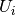the set of users that visited item, by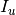the set of items visited by user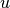, and by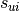the index of itemin the sequence of interactions of user. The frequency based time-weighted similarity function is defined by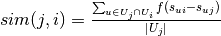, where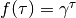is the time decaying function. For non-stationary data we sum only over users that visit item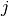before item, setting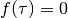if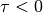. For stationary data the absolute value of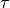is used. The score assigned to itemfor useris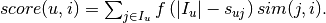The model is represented by the similarity scores. Since computing the model is time consuming, it is done periodically. Moreover, only the most similar items are stored for each item. When the prediction scores are computed for a particular user, all items visited by the user can be considered, including the most recent ones. Hence, the algorithm can be considered semi-online in that it uses the most recent interactions of the current user, but not of the other users. We note that the time decay function is used here to quantify the strength of connection between pairs of items depending on how closely are located in the sequence of a user, and not as a way to forget old data as in [Ding2005].

Sarwar2001
1. Sarwar, G. Karypis, J. Konstan, and J. Reidl. Item-based collaborative filtering recommendation algorithms. In Proc. WWW, pages 285–295, 2001.

Ding2005(1,2)
1. Ding and X. Li. Time weight collaborative filtering. In Proc. CIKM, pages 485–492. ACM, 2005.

Parameters
• gamma (double) – The constant used in the decay function. It shoud be set to 1 in offline and stationary experiments.

• direction (string) – Set to “forward” to consider the order of item pairs. Set to “both” when the order is not relevant.

• gamma_thresold (double) – Threshold to omit very small members when summing similarity. If the value of the decay function is smaller than the threshold, we omit the following members. Defaults to 0 (do not omit small members).

• num_of_neighbors (int) – The number of most similar items that will be stored in the model.

## alpenglow.experiments.OldFactorExperiment module¶

class alpenglow.experiments.OldFactorExperiment.OldFactorExperiment(dimension=10, begin_min=- 0.01, begin_max=0.01, learning_rate=0.05, regularization_rate=0.0, negative_rate=0.0)[source]

Deprecated, use FactorExperiment. This class implements an online version of the well-known matrix factorization recommendation model [Koren2009] and trains it via stochastic gradient descent. The model is able to train on implicit data using negative sample generation, see [X.He2016] and the negative_rate parameter.

Parameters
• dimension (int) – The latent factor dimension of the factormodel.

• begin_min (double) – The factors are initialized randomly, sampling each element uniformly from the interval (begin_min, begin_max).

• begin_max (double) – See begin_min.

• learning_rate (double) – The learning rate used in the stochastic gradient descent updates.

• regularization_rate (double) – The coefficient for the L2 regularization term.

• negative_rate (int) – The number of negative samples generated after each update. Useful for implicit recommendation.

## alpenglow.experiments.PersonalPopularityExperiment module¶

class alpenglow.experiments.PersonalPopularityExperiment.PersonalPopularityExperiment(**parameters)[source]

Recommends the item that the user has watched the most so far; in case of a tie, it falls back to global popularity. Running this model in conjunction with exclude_known == True is not recommended.

## alpenglow.experiments.PopularityExperiment module¶

class alpenglow.experiments.PopularityExperiment.PopularityExperiment(**parameters)[source]

Recommends the most popular item from the set of items seen so far.

## alpenglow.experiments.PopularityTimeframeExperiment module¶

class alpenglow.experiments.PopularityTimeframeExperiment.PopularityTimeframeExperiment(tau=86400)[source]

Time-aware version of PopularityModel, which only considers the last tau time interval when calculating popularities. Note that the time window ends at the timestamp of the last updating sample. The model does not take into consideration the timestamp of the sample for that the prediction is computed.

Parameters

tau (int) – The time amount to consider.

## alpenglow.experiments.PosSamplingFactorExperiment module¶

class alpenglow.experiments.PosSamplingFactorExperiment.PosSamplingFactorExperiment(dimension=10, begin_min=- 0.01, begin_max=0.01, base_learning_rate=0.2, base_regularization_rate=0.0, positive_learning_rate=0.05, positive_regularization_rate=0.0, negative_rate=40, positive_rate=3, pool_size=3000)[source]

This model implements an online, efficient technique that approximates the learning method of alpenglow.experiments.BatchAndOnlineFactorExperiment, using fewer update steps. Similarly to the online MF model (alpenglow.experiments.FactorExperiment), we only use a single iteration for the model in a temporal order. However, for each interaction, we generate not only negative but also positive samples. The positive samples are selected randomly from past interactions, i.e. we allow the model to re-learn the past. We generate positive_rate positive samples along with negative_rate negative samples, hence forevents, we only take (1+negative_rate+positive_rate)·t gradient steps.

The samples are not drawn uniformly from the past, but selected randomly from pool S with maximum size pool_size. This avoids oversampling interactions that happened at the beginning of the data set. More specifically, for each observed new training instance, we

• update the model by positive_rate samples from pool S,

• delete the selected samples from pool S if it already reached pool_size,

• and add the new instance positive_rate times to the pool.

For more details, see [frigo2017online] .

frigo2017online

Frigó, E., Pálovics, R., Kelen, D., Kocsis, L., & Benczúr, A. (2017). “Online ranking prediction in non-stationary environments.” Section 3.5.

Parameters
• dimension (int) – The latent factor dimension of the factormodel.

• begin_min (double) – The factors are initialized randomly, sampling each element uniformly from the interval (begin_min, begin_max).

• begin_max (double) – See begin_min.

• negative_rate (int) – The number of negative samples generated after each update. Useful for implicit recommendation.

• positive_rate (int) – The number of positive samples generated for each update.

• pool_size (int) – The size of pool for generating positive samples. See the article for details.

• base_learning_rate (double) – The learning rate used in the stochastic gradient descent updates for the original positive sample and the generated negative samples.

• base_regularization_rate (double) – The coefficient for the L2 regularization term.

• positive_learning_rate (double) – The learning rate used in the stochastic gradient descent updates for the generated positive samples.

• positive_regularization_rate (double) – The coefficient for the L2 regularization term.

## alpenglow.experiments.SvdppExperiment module¶

class alpenglow.experiments.SvdppExperiment.SvdppExperiment(begin_min=- 0.01, begin_max=0.01, dimension=10, learning_rate=0.05, negative_rate=20, use_sigmoid=False, norm_type='exponential', gamma=0.8, user_vector_weight=0.5, history_weight=0.5)[source]

This class implements an online version of the SVD++ model [Koren2008] The model is able to train on implicit data using negative sample generation, see [X.He2016] and the negative_rate parameter. We apply a decay on the user history, the weight of the older items is smaller.

Koren2008
1. Koren, “Factorization Meets the Neighborhood: A Multifaceted Collaborative Filtering Model,” Proc. 14th ACM SIGKDD Int’l Conf. Knowledge Discovery and Data Mining, ACM Press, 2008, pp. 426-434.

Parameters
• begin_min (double) – The factors are initialized randomly, sampling each element uniformly from the interval (begin_min, begin_max).

• begin_max (double) – See begin_min.

• dimension (int) – The latent factor dimension of the factormodel.

• learning_rate (double) – The learning rate used in the stochastic gradient descent updates.

• negative_rate (int) – The number of negative samples generated after each update. Useful for implicit recommendation.

• norm_type (string) – Normalization variants.

• gamma (double) – The constant in the decay function.

• user_vector_weight (double) – The user is modeled with a sum of a user vector and a combination of item vectors. The weight of the two part can be set using these parameters.

• history_weight (double) – See user_vector_weight.

## alpenglow.experiments.TransitionProbabilityExperiment module¶

class alpenglow.experiments.TransitionProbabilityExperiment.TransitionProbabilityExperiment(mode='normal')[source]

A simple algorithm that focuses on the sequence of items a user has visited is one that records how often users visited item i after visiting another item j. This can be viewed as particular form of the item-to-item nearest neighbor with a time decay function that is non-zero only for the immediately preceding item. While the algorithm is more simplistic, it is fast to update the transition fre- quencies after each interaction, thus all recent information is taken into account.

Parameters

mode (string) – The direction of transitions to be considered, possible values: normal, inverted, symmetric.